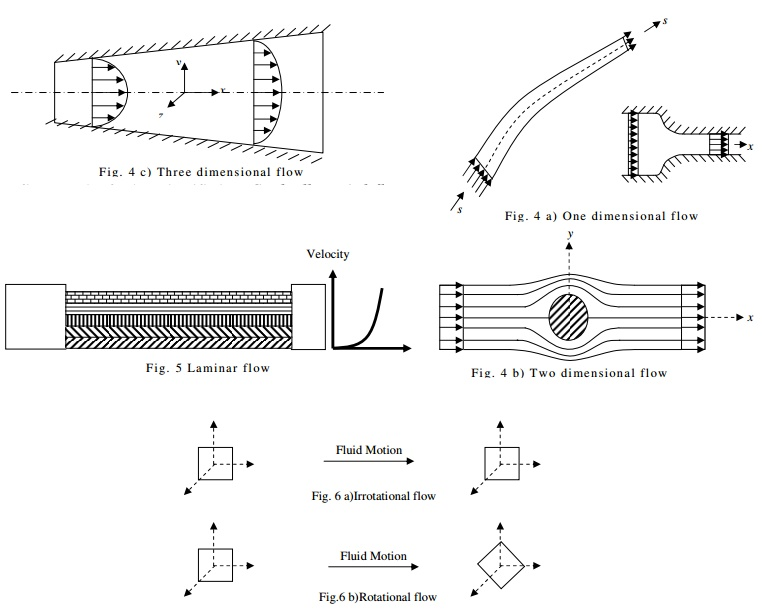Home | | Mechanics of Fluids | Fluid Kinematics And Dynamics: Classification of Flows

# Fluid Kinematics And Dynamics: Classification of Flows1. Steady and unsteady flows: 2. Uniform and non-uniform flows: 3. One, two and three dimensional flows: 4. Laminar and Turbulent flows: 5. Compressible and Incompressible flows: 6. Rotational and Irrotational flows: 7. Critical, Sub-critical and Super-critical flows:

Classification of Flows:

A flow is said to be steady if the properties (P) of the fluid and flow do not change

? ( P) = 0 ?t

with time (t) at any section or point in a fluid flow.

A flow is said to be unsteady if the properties (P) of the fluid and flow change with

? ( P) ? 0 ?t

time (t) at any section or point in a fluid flow.

Eg: Flow observed at a dam section during rainy season, wherein, there will be lot of inflow with which the flow properties like depth, velocity etc.. will change at the dam section over a period of time representing it as unsteady flow.

2. Uniform and non-uniform flows:

A flow is said to be uniform if the properties (P) of the fluid and flow do not change (with direction) over a length of flow considered along the flow at any instant.A flow is said to be non-uniform if the properties (P) of the fluid and flow change (with direction) over a length of flow considered along the flow at any instant.

Eg: Flow observed at any instant, at the dam section during rainy season, wherein, the flow varies from the top of the overflow section to the foot of the dam and the flow properties like depth, velocity etc., will change at the dam section at any instant between two sections, representing it as non-uniform flow.

Consider a fluid flow as shown above in a channel. The flow is said to be steady at sections 1 and 2 as the flow does not change with respect to time at the respective sections (y1=y2 and v1=v2 ).

The flow between sections 1 and 2 is said to be uniform as the properties does not change between the sections at any instant (y1 =y2 and v1 = v2).

The flow between sections 2 and 3 is said to be non-uniform flow as the properties vary over the length between the sections.Non-uniform flow can be further classified as Gradually varied flow and Rapidly varied flow. As the name itself indicates, Gradually varied flow is a non-uniform flow wherein the flow/fluid properties vary gradually over a long length (Eg: between sections 2 and 3).

Rapidly varied flow is a non-uniform flow wherein the flow/fluid properties vary rapidly within a very short distance. (Eg: between sections 4 and 5).

(Sections 4 and 5).

3. One, two and three dimensional flows:

Flow is said to be direction and will one-dimensional if the properties vary only along one axis / be constant with respect to other two directions of a three-Flow is said to be two-dimensional if the properties vary only along two axes / directions and will be constant with respect to other direction of a three-dimensional axis system.

Flow is said to be three-dimensional if the properties vary along all the axes / directions of a three-dimensional axis system.

4. Laminar and Turbulent flows:

When the flow occurs like sheets or laminates and the fluid elements flowing in a layer does not mix with other layers, then the flow is said to be laminar. The Reynolds number (Re) for the flow will be less than 2000.When the flow velocity increases, the sheet like flow gets mixed up and the fluid elements mix with other layers there by causing turbulence. There will be eddy currents generated and flow reversal takes place. This flow is said to be Turbulent.The Reynolds number for the flow will be greater than 4000.

For flows with Reynolds number between 2000 to 4000 is said to be transition flow.

5. Compressible and Incompressible flows:

Flow is said to be Incompressible if the fluid density does not change (constant) along the flow direction and is Compressible if the fluid density varies along the flow direction

? = Constant (incompressible) and ? ? Constant (compressible)

6. Rotational and Irrotational flows:

Flow is said to be Rotational if the fluid elements does not rotate about their own axis as they move along the flow and is Rotational if the fluid elements rotate along their axis as they move along the flow direction.7. Critical, Sub-critical and Super-critical flows:

Froude's Number

It is the ratio of the inertia forces to gravity forces and mathematicallywhere Fe=Froudes No, V is the flow velocity and d is the hydraulic mean gd depth given by

d-A/T, A is the flow cross-sectional area and T is the top width.T

If the Foude's number is ONE, the flow is critical, Less than ONE, Sub-critical and Greater than ONE, Super-critical.

Rate of flow or Discharge (Q):

Rate of flow or discharge is said to be the quantity of fluid flowing per second across a section of a flow. Rate of flow can be expressed as mass rate of flow or volume rate of flow. Accordingly

Mass rate of flow = Mass of fluid flowing across a section / time Rate of flow = Volume of fluid flowing across a section / time

Types of lines

Path Line: It is the path traced by a fluid particle over a period of time during its motion along the fluid flow.Eg: Path traced by an ant coming out from its dwelling

Study Material, Lecturing Notes, Assignment, Reference, Wiki description explanation, brief detail
Civil - Mechanics Of Fluids - Fluid Kinematics And Dynamics : Fluid Kinematics And Dynamics: Classification of Flows |

Related Topics